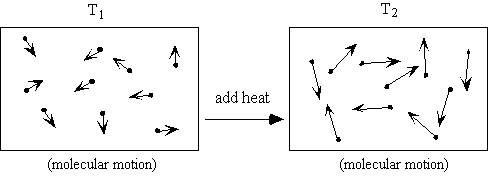# First law of thermodynamics incorporates the law ofa) conservation of energyb)conservation of heatc)conservation of workd) equivalence of heat and workwhich ones and why.?

8 years ago

## First Law of Thermodynamics(VW, S & B: 2.6)

1. ### There exists for every system a property called energy.E = internal energy (arising from molecular motion - primarily a function of temperature) + kinetic energy + potential energy + chemical energy

1. Defines a useful property called "energy".

2. The two new terms in the equation (compared to what you have seen in physics and dynamics, for example) are the internal energy and the chemical energy. For most situtations in this class, we will neglect the chemical energy.

3. Let’s focus on the internal energy, u. It is associated with the random or disorganized motion of the particles.u is a function of the state of the system. Thus u = u (p, T), or u = u (p, v), or u = u(v,T). Recall that for pure substances the entire state of the system is specified if anytwoproperties are specified. We will discuss the equations that relate the internal energy to these other variables later in the class.

## DE = Q - W (units are Joules)

Thanks & Regards
Rinkoo Gupta